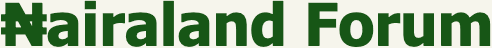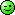#Welcome, Guest: Join Nairaland / LOGIN! / Trending / Recent / New
Stats: 2,422,245 members, 5,434,089 topics. Date: Friday, 21 February 2020 at 07:50 AM

## Mathematicians, Check In Here! - Education - Nairaland

 Mathematicians, Check In Here! by geraldinai(f): 1:03am On Feb 14 Mathematicians in the house, please I need help with this.Solve for x3cos(x) + 2sin2(x) =0Thanks. Re: Mathematicians, Check In Here! by Martinez39s(m): 4:06am On Feb 14 geraldinai:Mathematicians in the house, please I need help with this.Solve for x3cos(x) + 2sin2(x) =0Thanks. SOLUTION 3Cos x + 2Sin² x = 0Given that Sin² x = 1 – Cos² x3Cos x + 2Sin² x = 3Cosx + 2(1 – Cos² x) = –2Cos² x + 3Cos x + 2–2Cos² x + 3Cos x + 2 = 0 is an equation quadrant in form. It is quadratic in Cos x. By substituting u for Cos x, we have –2u²+ 3u + 2 = 0The quadratic formula yields two answers: u = –0.5 & u = 2. u = 2 is not in the range of the cosine function so we discard it. The solutions you seek are solutions of the equation Cos x = –0.5If n is any integer then the solutions are x = 120° + n360° & x = 240° + n360° In radians, x = (2π/3) + 2πn & x = (4π/3) + 2πn4 Likes Re: Mathematicians, Check In Here! by geraldinai(f): 11:41pm On Feb 14 Martinez39s: SOLUTION 3Cos x + 2Sin² x = 0Given that Sin² x = 1 – Cos² x–2Cos² x + 3Cos x + 2 = 0 is an equal quadrant in form. It is quadratic in Cos x. By substituting u for Cos x, we have The quadratic formula yields two answers: u = –0.5 & u = 2. u = 2 is not in the range of the cosine function so we discard it. The solutions you seek are solutions of the equation Cos x = –0.5If n is any integer then the solutions are x = 120° + n360° & x = 240° + n360° In radians, x = (2π/3) + 2πn & x = (4π/3) + 2πnWow! Well explained. Thanks bro